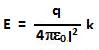## Sunday, January 24, 2010

### Irodov Problem 3.9Consider an infinitesimally thin section of the ring that subtends and angle at the center of the circle. The point in question, O, is located exactly above the center at a height l from the plane of the ring. The charge in the infinitesimally small section isThe electric field due to the infinitesimally small section of ring will be equal to,From the figure we can see that,Hence, the electric field is given by,When l much larger than r, (4) simply becomes,In other words it acts like a point charge.

Clearly the electric field is zero at the center of the ring (l=0) and also zero when l is very very large. Hence, there must be a value of l for which the electric field is maximum. This value of l can be obtained by setting the first derivative of E to zero as follows,From (5) and (4) we have the maximum possible value of E as,1.2.